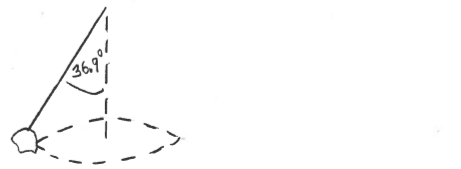Problem: A small rock is tied to a light string set it motion in a horizontal circle with constant speed. The string is 8.00 m long and makes a constant angle of 36.9° with the vertical direction. The tension in the string is 6.20 N.What is the radius r of the circular path of the rock?

FREE Expert Solution
94% (373 ratings)
Problem Details

A small rock is tied to a light string set it motion in a horizontal circle with constant speed. The string is 8.00 m long and makes a constant angle of 36.9° with the vertical direction. The tension in the string is 6.20 N.

What is the radius r of the circular path of the rock?